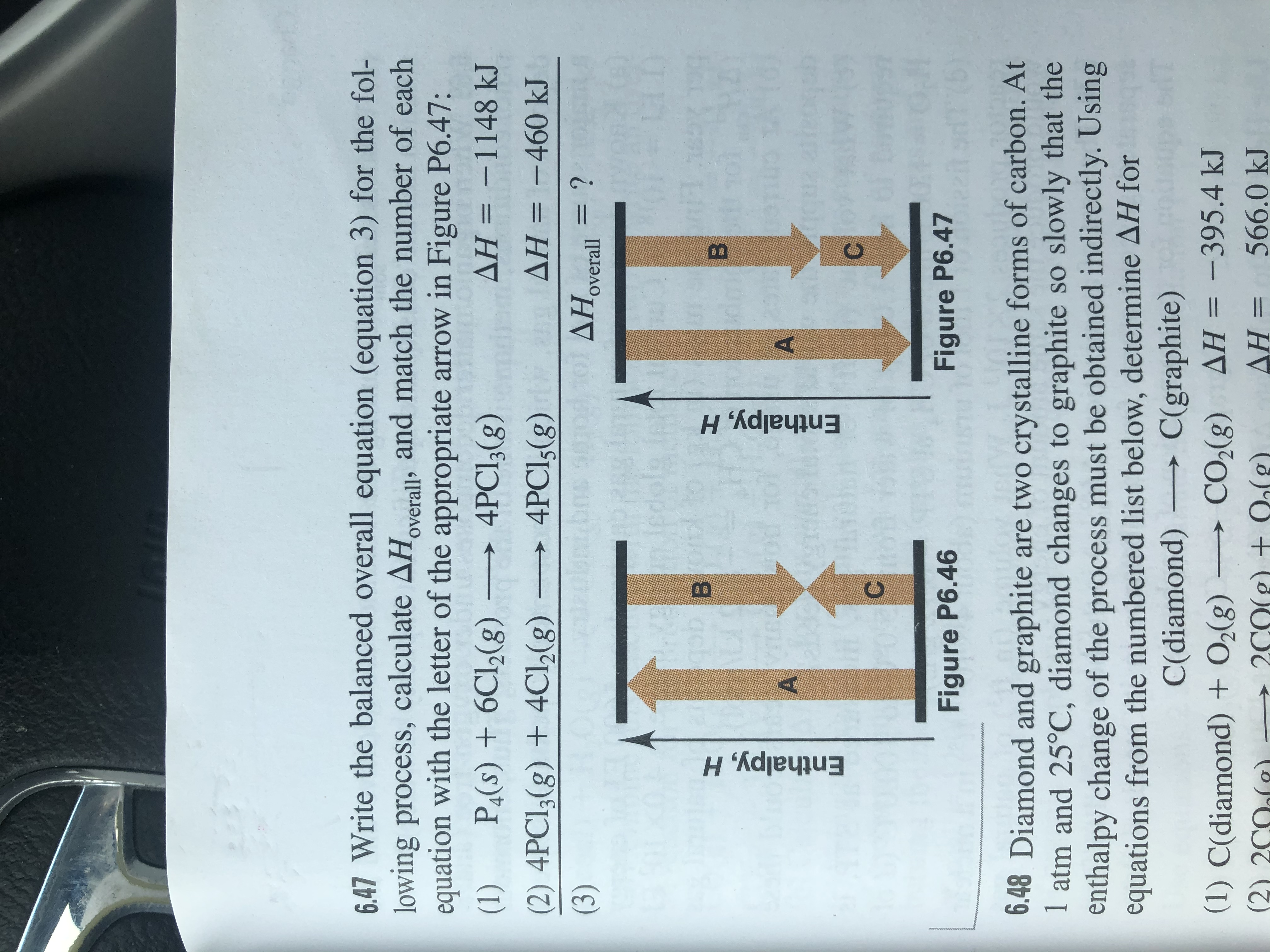# Write the balanced overall equation (Equation 3) for the following process, calculate ΔHoverall, and match the number of each equation with the letter of the appropriate arrow in Figure P6.47. (1) P4 (s) + 6 Cl2 (g) → 4 PCl3 (g) ΔH = -1148 kJ (2) 4 PCl3 (g) + 4 Cl2 (g) → 4 PCl5 (g) ΔH = - 460 kJ